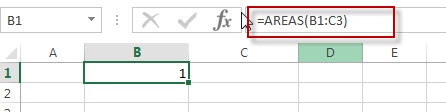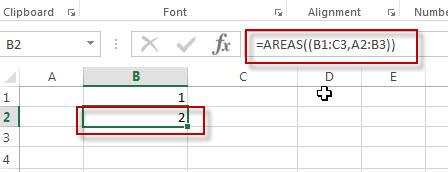# Excel Areas Function

This post will guide you how to use Excel AREAS function with syntax and examples in Microsoft excel.

### Description

The Excel AREAS function returns the number of ranges in a reference.

The AREAS function is a build-in function in Microsoft Excel and it is categorized as a Lookup and Reference Function.

The AREAS function is available in Excel 2016, Excel 2013, Excel 2010, Excel 2007, Excel 2003, Excel XP, Excel 2000, Excel 2011 for Mac.

### Syntax

The syntax of the AREAS function is as below:

=AREAS (reference)

Where the AREAS function arguments are:
Reference -This is a required argument. A Excel cell reference or A range reference, it also can be a multiple ranges.

### Example

The below examples will show you how to use Excel AREAS Lookup and Reference Function to return the number of ranges in a cell reference.

#1  Formula: =AREAS(B1:C3)Note: the above excel formula will return the number of ranges in B1:C3 range cells.

#2 Formula: =AREAS((B1:C3,A2:B3))